# 2018 GEOMETR Y boot camp Circles Geometric Measurement

• Slides: 62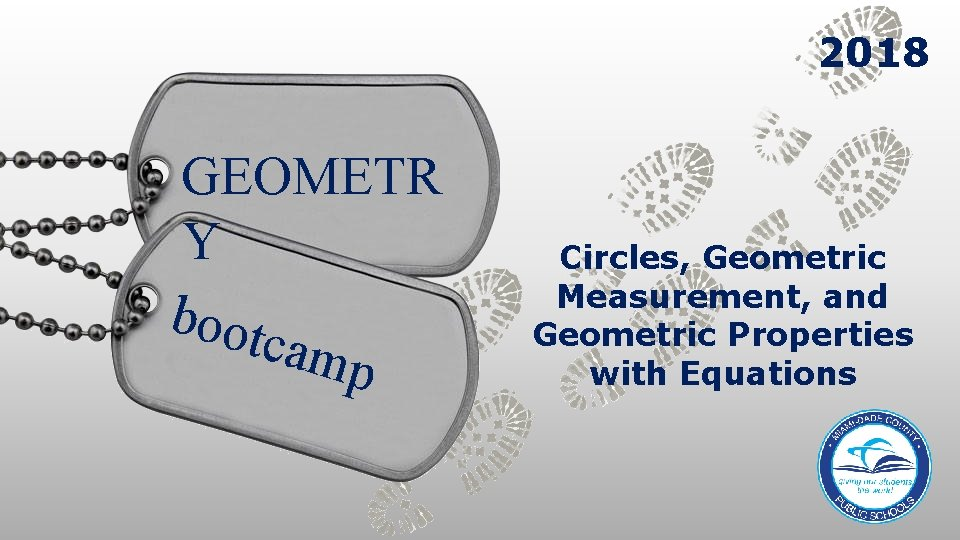2018 GEOMETR Y boot camp Circles, Geometric Measurement, and Geometric Properties with Equations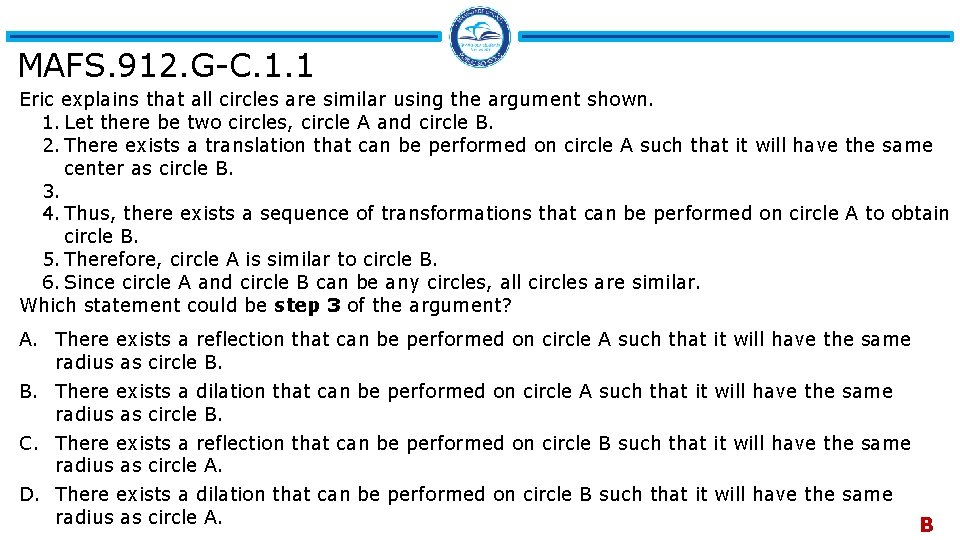MAFS. 912. G-C. 1. 1 Eric explains that all circles are similar using the argument shown. 1. Let there be two circles, circle A and circle B. 2. There exists a translation that can be performed on circle A such that it will have the same center as circle B. 3. 4. Thus, there exists a sequence of transformations that can be performed on circle A to obtain circle B. 5. Therefore, circle A is similar to circle B. 6. Since circle A and circle B can be any circles, all circles are similar. Which statement could be step 3 of the argument? A. There exists a reflection that can be performed on circle A such that radius as circle B. B. There exists a dilation that can be performed on circle A such that it radius as circle B. C. There exists a reflection that can be performed on circle B such that radius as circle A. D. There exists a dilation that can be performed on circle B such that it radius as circle A. it will have the same B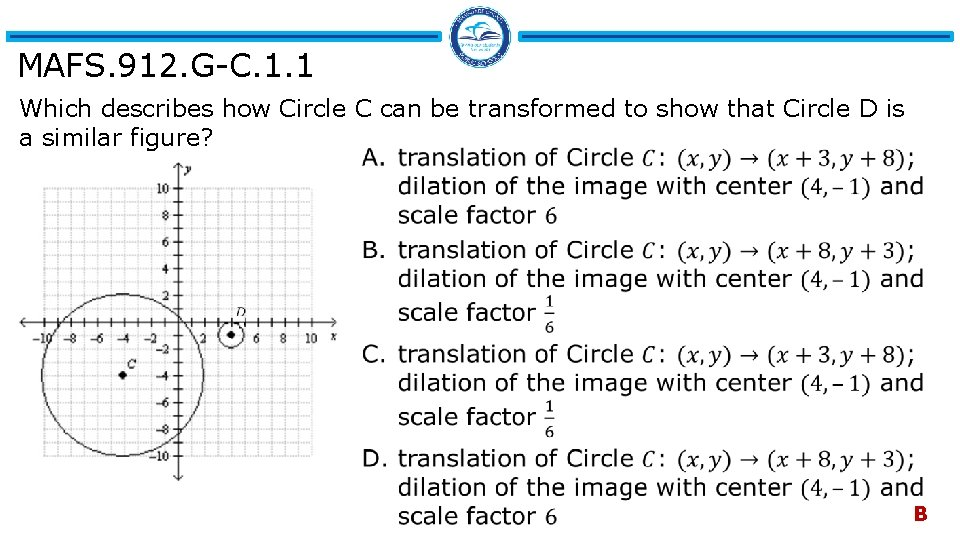MAFS. 912. G-C. 1. 1 Which describes how Circle C can be transformed to show that Circle D is a similar figure? B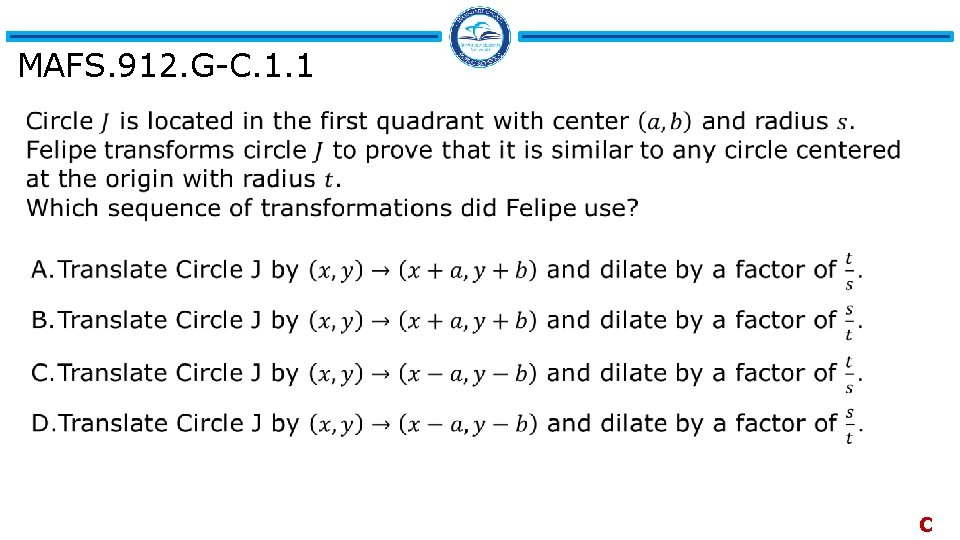MAFS. 912. G-C. 1. 1 C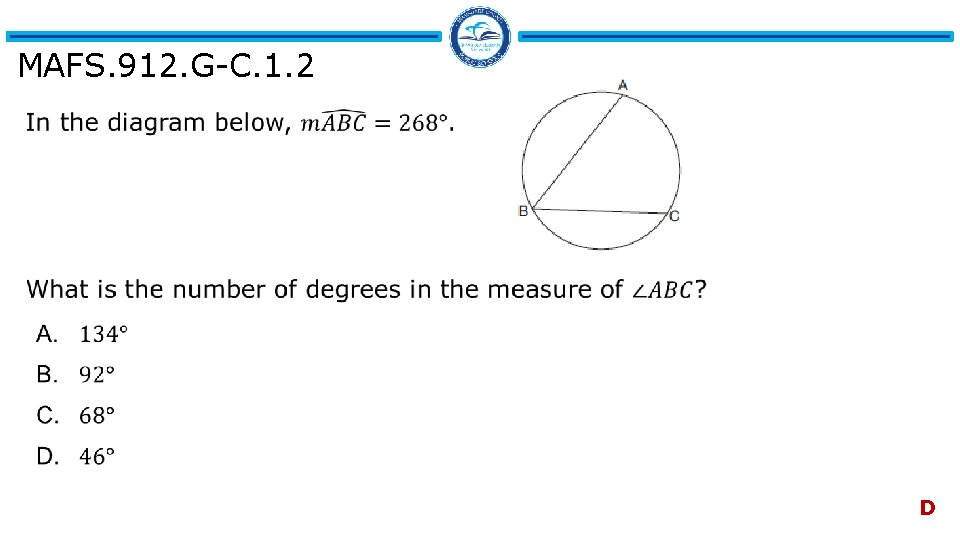MAFS. 912. G-C. 1. 2 D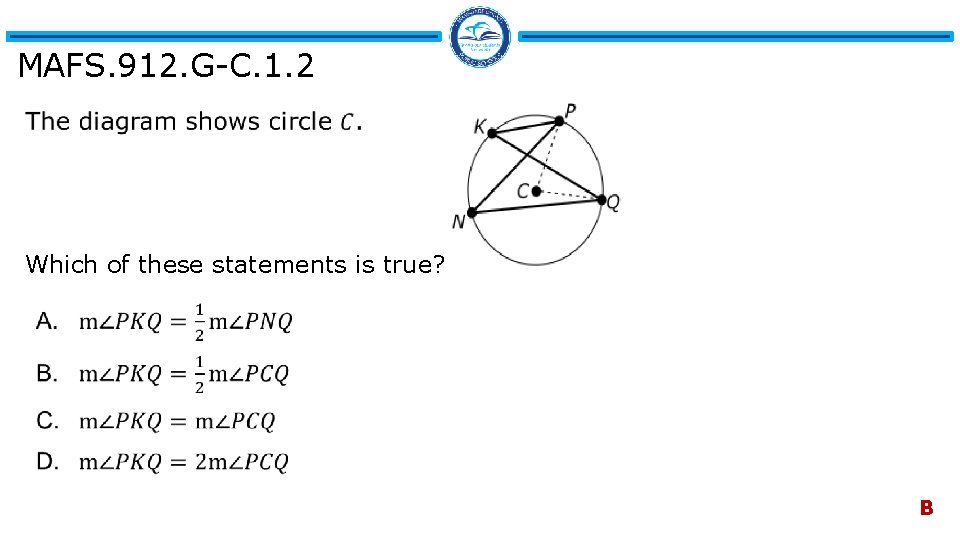MAFS. 912. G-C. 1. 2 Which of these statements is true? B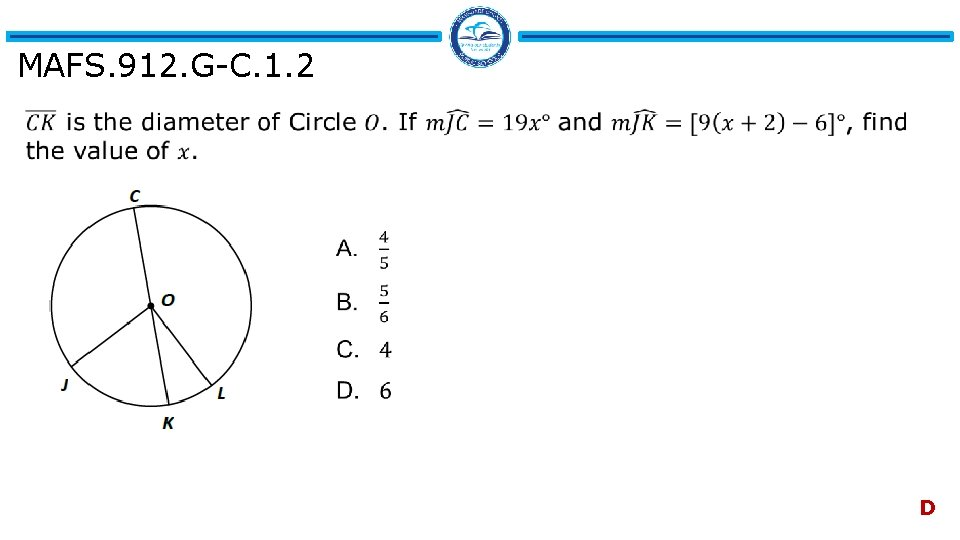MAFS. 912. G-C. 1. 2 DMAFS. 912. G-C. 1. 2 Maggie and Wei are measuring the distance across a circular fountain indirectly as shown in the diagram. Enter your answer in the box.MAFS. 912. G-C. 1. 2 Points A, B, and D lie on circle C. ° °MAFS. 912. G-C. 1. 2 The figure below shows concentric circles, both centered at O. • Chord XY is tangent to the smaller circle. • The radius of the larger circle is 15 cm. • The radius of the smaller circle is 12 cm. What is the length of chord XY? A. 27 cm B. 24 cm C. 18 cm D. 10 cm CMAFS. 912. G-C. 1. 3 A. 20 B. 25 C. 38 D. 40 B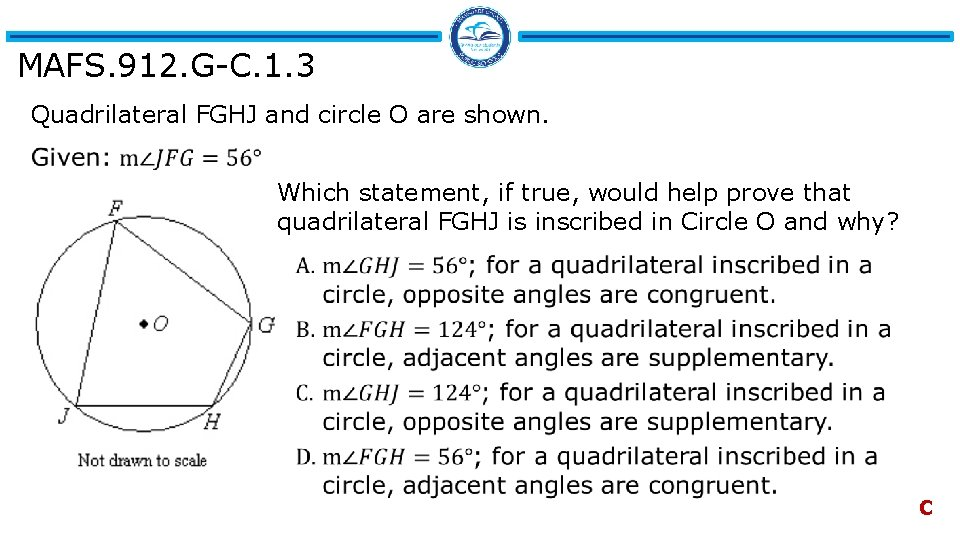MAFS. 912. G-C. 1. 3 Quadrilateral FGHJ and circle O are shown. Which statement, if true, would help prove that quadrilateral FGHJ is inscribed in Circle O and why? C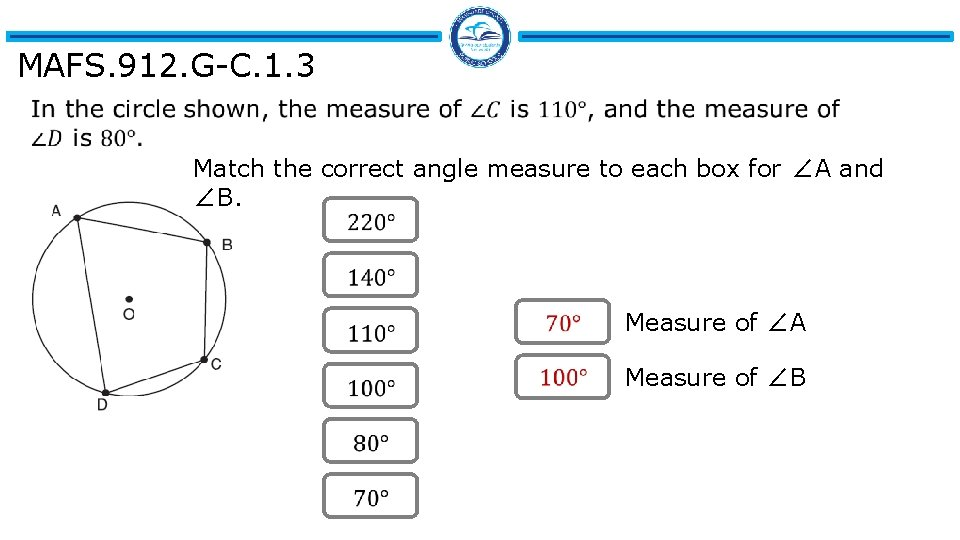MAFS. 912. G-C. 1. 3 Match the correct angle measure to each box for ∠A and ∠B. Measure of ∠A Measure of ∠BMAFS. 912. G-C. 1. 3 Select all statements that are valid when a triangle is inscribed in a circle. q the circle is circumscribed about the triangle. q the perpendicular bisectors of the triangle may be constructed to find the center of the circle. q the center of the circumscribed circle must always be in the interior of the triangle. q the vertices of the triangle are equidistant from the center of the circle. q the triangle must be isosceles.MAFS. 912. G-C. 2. 5 Points A, B, D, and E lie on circle C. What is the length of arc ADB? A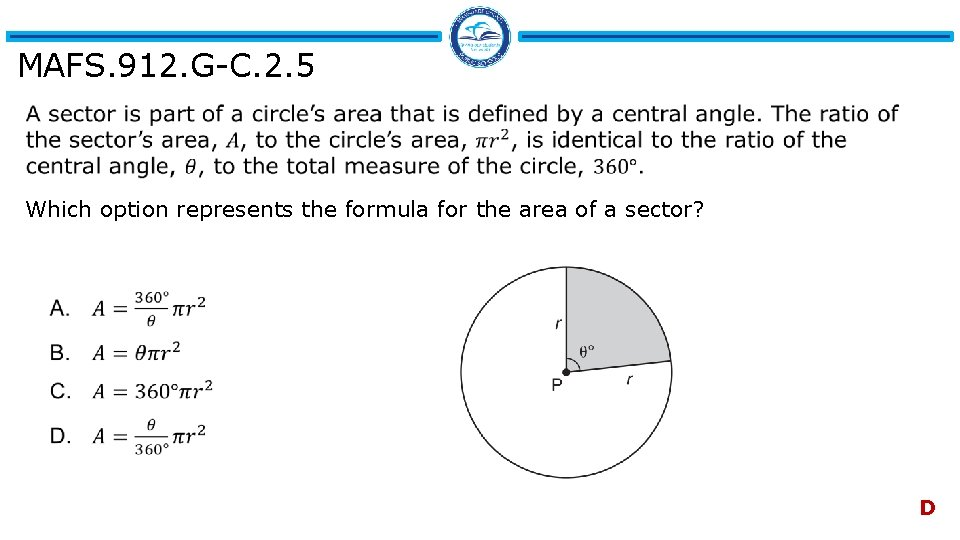MAFS. 912. G-C. 2. 5 Which option represents the formula for the area of a sector? D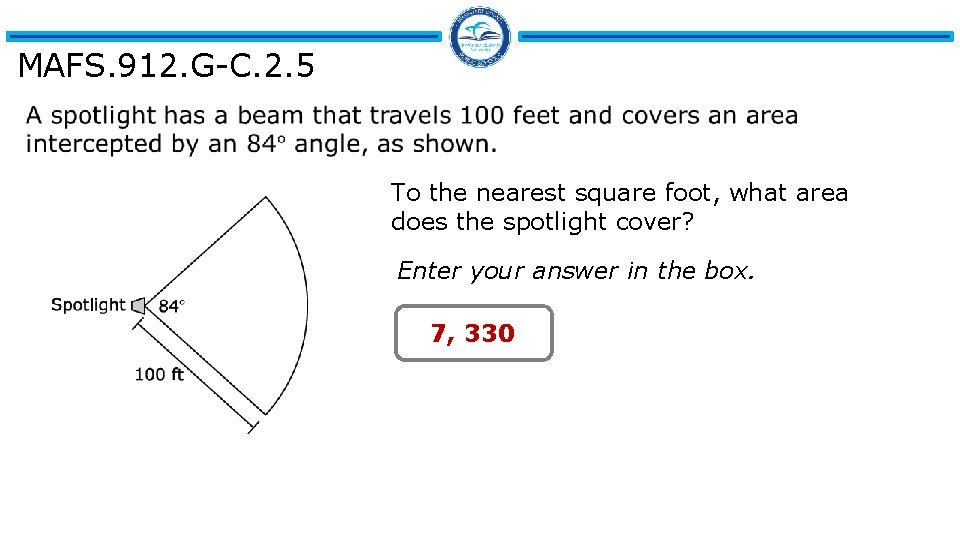MAFS. 912. G-C. 2. 5 To the nearest square foot, what area does the spotlight cover? Enter your answer in the box. 7, 330MAFS. 912. G-C. 2. 5 Drag and drop each arc length to its subtended central angle. Subtended Central Angle Arc Length in radiansMAFS. 912. G-GMD. 1. 1 To find the formula for the area of a circle, the circle can be cut into “slices, ” as indicated below. Which statement best describes the process being used? CMAFS. 912. G-GMD. 1. 1 The cylinder and the cone shown below have the same height, and their bases have the same radius. D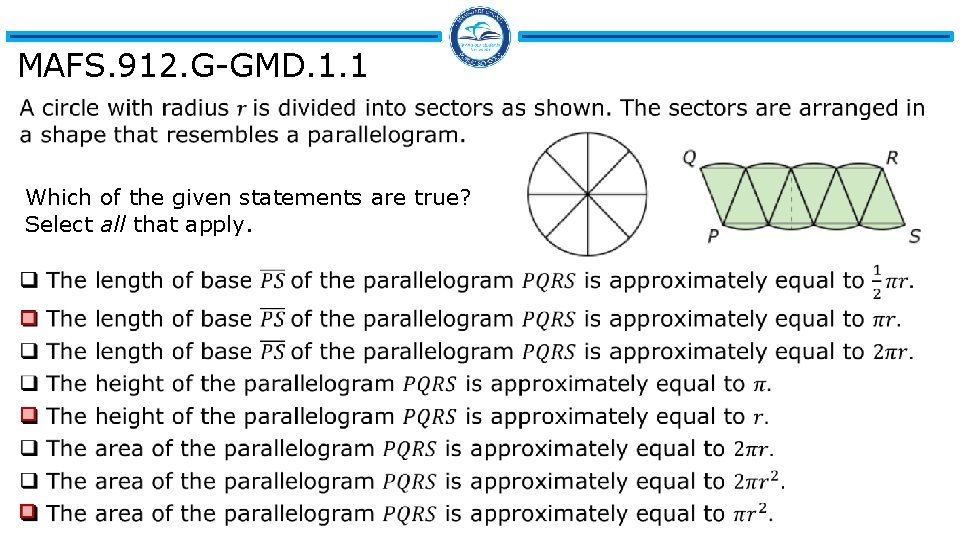MAFS. 912. G-GMD. 1. 1 Which of the given statements are true? Select all that apply.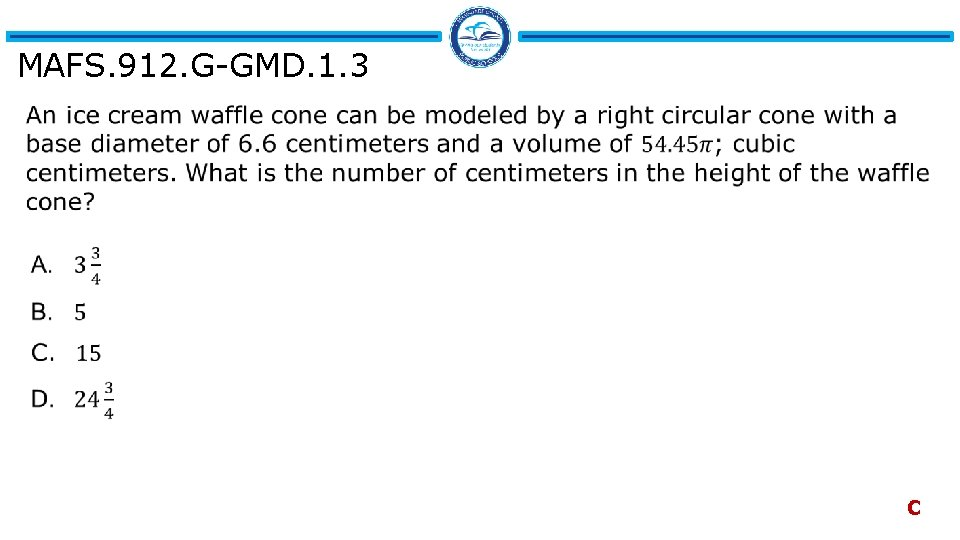MAFS. 912. G-GMD. 1. 3 C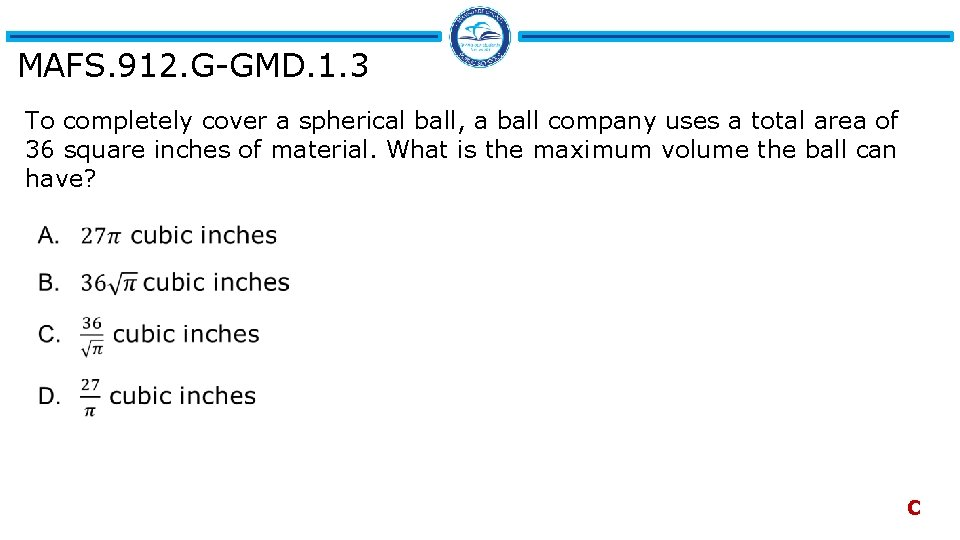MAFS. 912. G-GMD. 1. 3 To completely cover a spherical ball, a ball company uses a total area of 36 square inches of material. What is the maximum volume the ball can have? CMAFS. 912. G-GMD. 1. 3 A regular pyramid has a square base. The perimeter of the base is 36 inches and the height of the pyramid is 15 inches. What is the volume of the pyramid in cubic inches? A. 180 B. 405 C. 540 D. 1215 BMAFS. 912. G-GMD. 1. 3 A soft drink company wants to increase the volume of the cylindrical can they sell soft drinks in by 25%. The company wants to keep the 5 -inch height of the can the same. The radius of the can is currently 2. 5 inches. Approximately how much should the radius of the can be increased? A. 0. 245 inches B. 0. 250 inches C. 0. 278 inches D. 0. 295 inches DMAFS. 912. G-GMD. 1. 3 Trevon is shopping for an art project for his class of third graders. He wants each of his 30 students to have a ball of modeling clay 10 centimeters in diameter. The modeling clay is sold in cylindrical rolls that are 15 centimeters long and 4 centimeters in diameter. How many rolls of clay does he need to buy? Enter your answer in the box. 84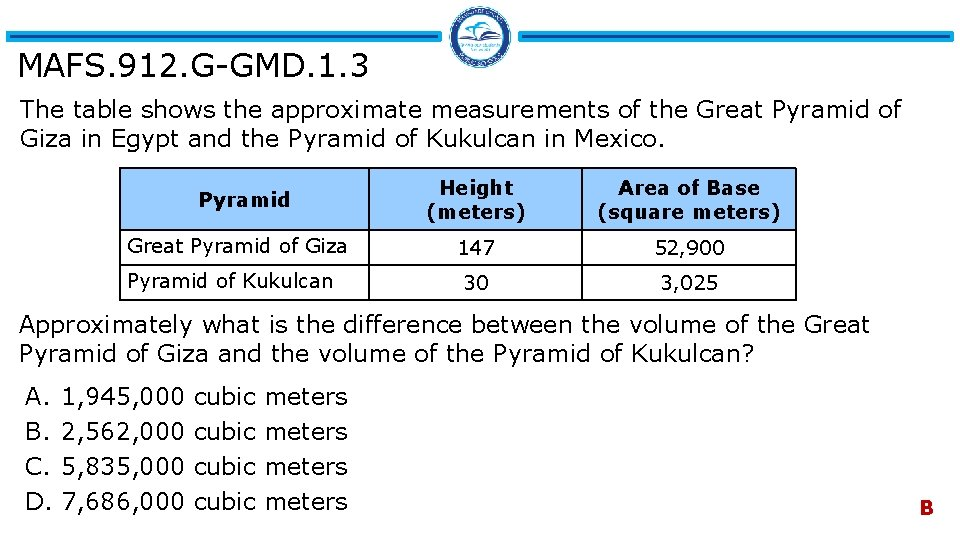MAFS. 912. G-GMD. 1. 3 The table shows the approximate measurements of the Great Pyramid of Giza in Egypt and the Pyramid of Kukulcan in Mexico. Pyramid Great Pyramid of Giza Pyramid of Kukulcan Height (meters) Area of Base (square meters) 147 52, 900 30 3, 025 Approximately what is the difference between the volume of the Great Pyramid of Giza and the volume of the Pyramid of Kukulcan? A. B. C. D. 1, 945, 000 2, 562, 000 5, 835, 000 7, 686, 000 cubic meters B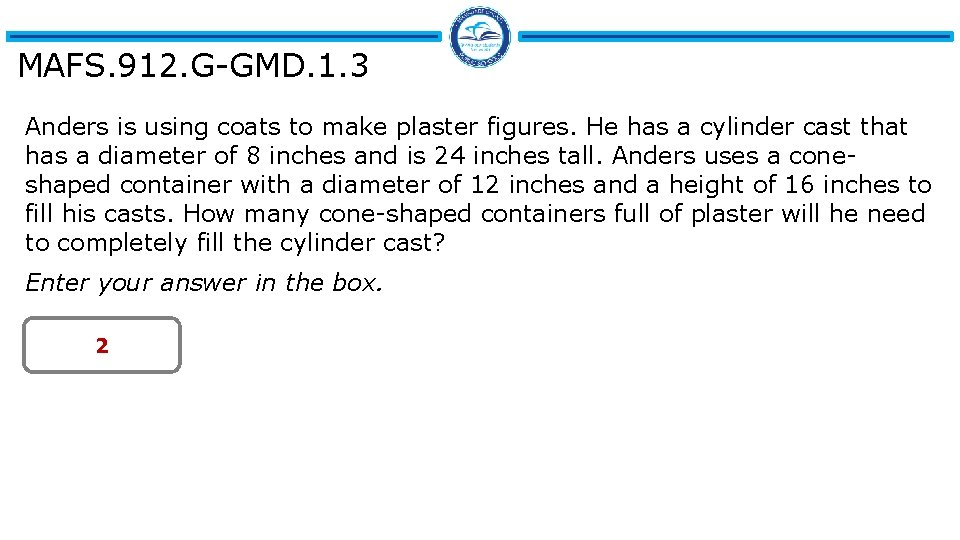MAFS. 912. G-GMD. 1. 3 Anders is using coats to make plaster figures. He has a cylinder cast that has a diameter of 8 inches and is 24 inches tall. Anders uses a coneshaped container with a diameter of 12 inches and a height of 16 inches to fill his casts. How many cone-shaped containers full of plaster will he need to completely fill the cylinder cast? Enter your answer in the box. 2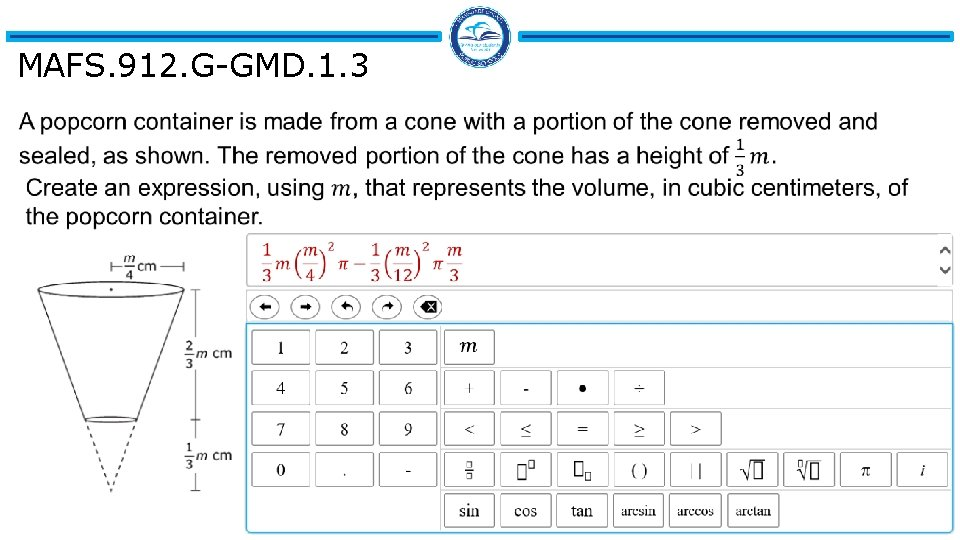MAFS. 912. G-GMD. 1. 3MAFS. 912. G-GMD. 2. 4 The figure shows a plane slicing through a cone. The plane is neither parallel nor perpendicular to the base of the cone, and the plane does not intersect the base of the cone. What is the shape of the cross section created by the slice? A. Circle B. Ellipse C. Parabola D. Triangle BMAFS. 912. G-GMD. 2. 4 A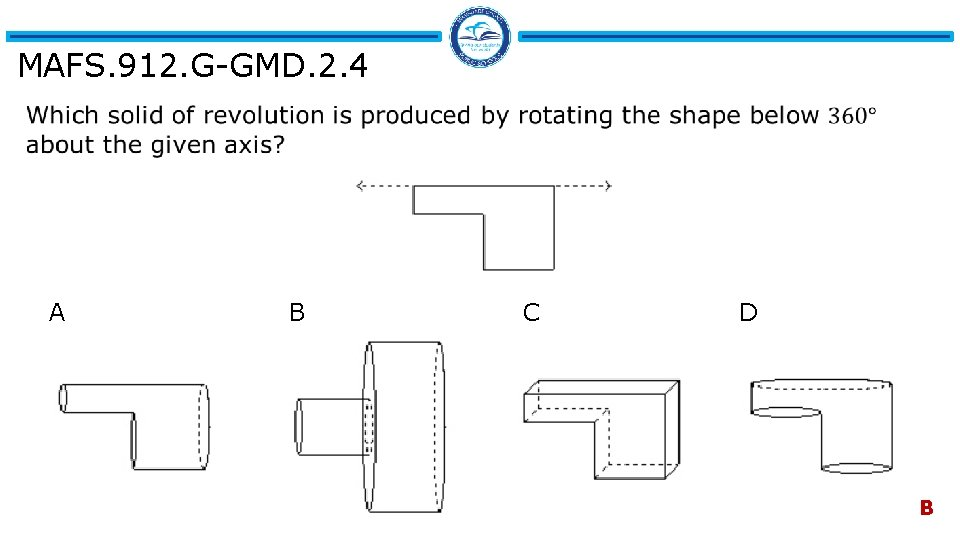MAFS. 912. G-GMD. 2. 4 A B C D B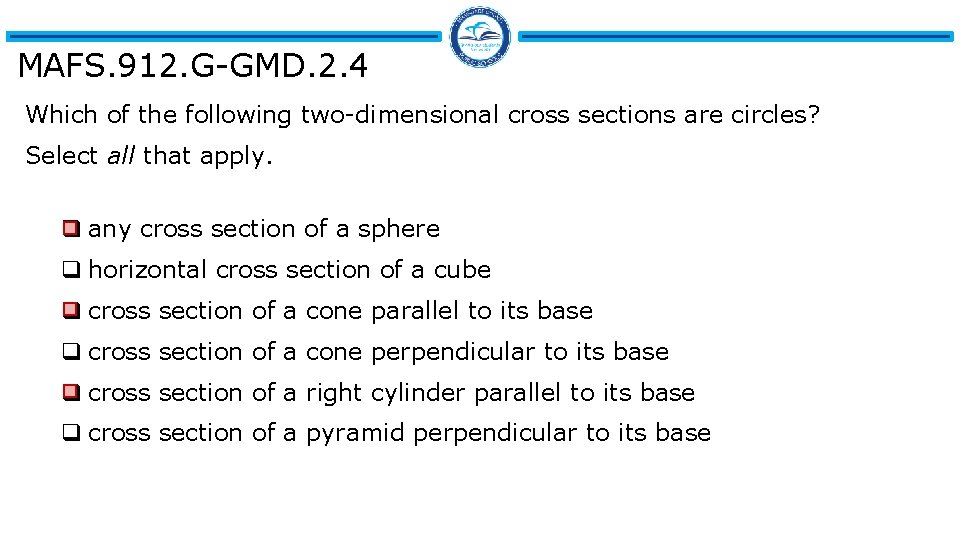MAFS. 912. G-GMD. 2. 4 Which of the following two-dimensional cross sections are circles? Select all that apply. q any cross section of a sphere q horizontal cross section of a cube q cross section of a cone parallel to its base q cross section of a cone perpendicular to its base q cross section of a right cylinder parallel to its base q cross section of a pyramid perpendicular to its base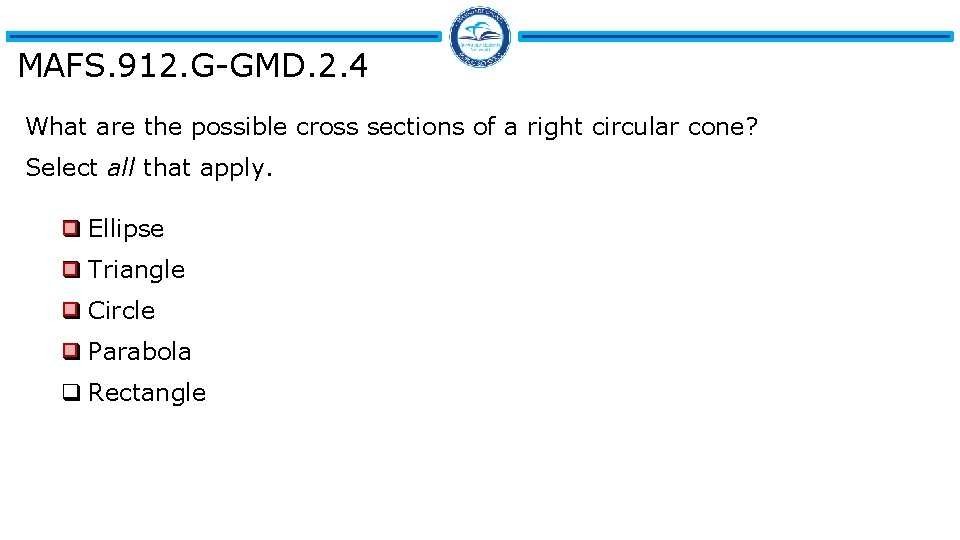MAFS. 912. G-GMD. 2. 4 What are the possible cross sections of a right circular cone? Select all that apply. q Ellipse q Triangle q Circle q Parabola q RectangleMAFS. 912. G-GMD. 2. 4 A triangle has vertices at (0, 0), (0, 9), and (4, 0). A. a pyramid with a square base of 4 units by 4 units and a height of 9 units B. a pyramid with a square base of 9 units by 9 units and a height of 4 units C. a cone with a diameter of 8 units and a height of 9 units D. a cone with a diameter of 18 units and a height of 4 units DMAFS. 912. G-GPE. 1. 1 A circle has its center at (− 2, 3) and point (4, 6) is on its circumference. What is the correct written equation of the circle? DMAFS. 912. G-GPE. 1. 1 Write the equation of a circle with a radius of 5 units and a center at ( -3, 4)?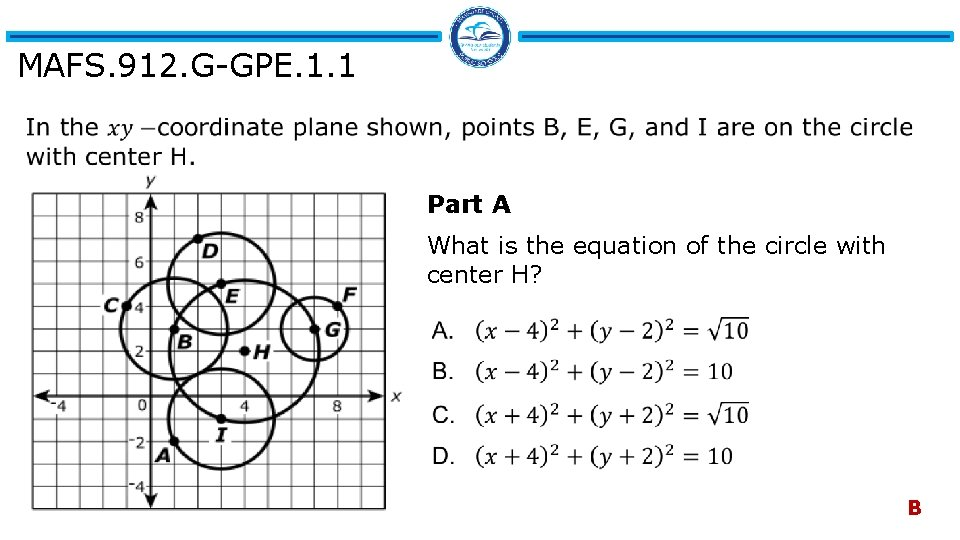MAFS. 912. G-GPE. 1. 1 Part A What is the equation of the circle with center H? B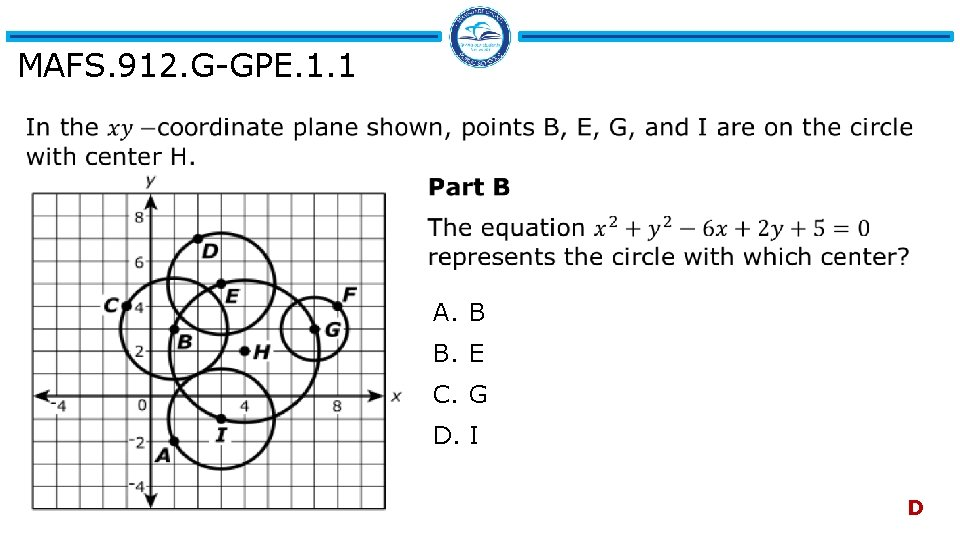MAFS. 912. G-GPE. 1. 1 A. B B. E C. G D. I D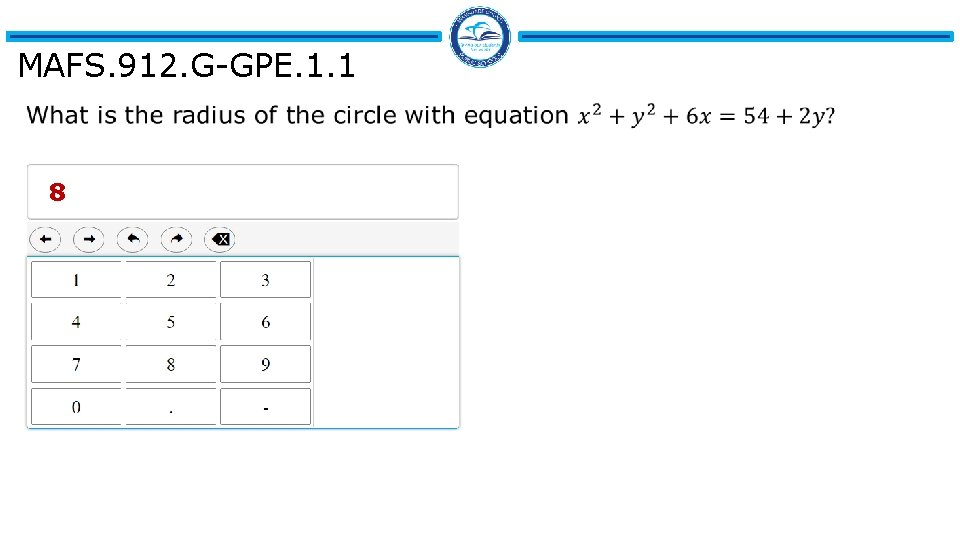MAFS. 912. G-GPE. 1. 1 8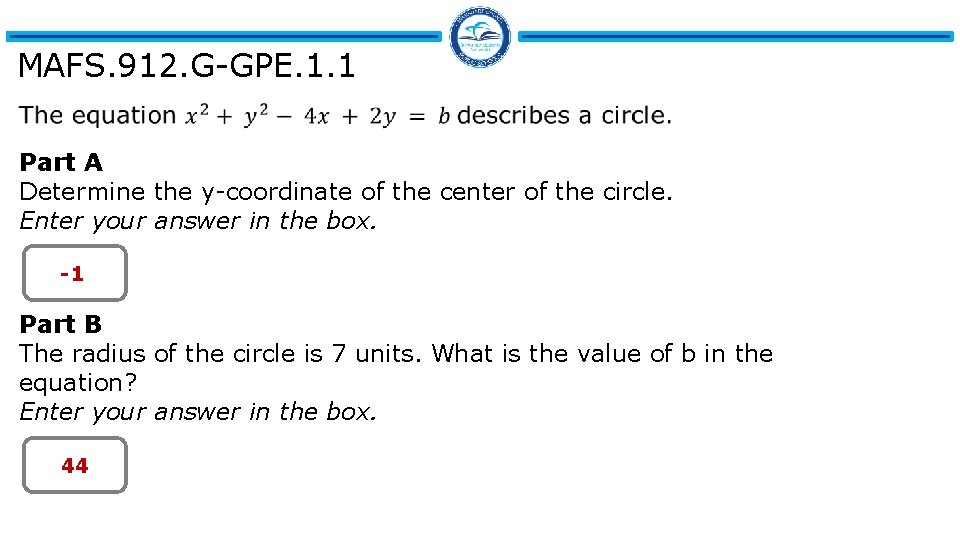MAFS. 912. G-GPE. 1. 1 Part A Determine the y-coordinate of the center of the circle. Enter your answer in the box. -1 Part B The radius of the circle is 7 units. What is the value of b in the equation? Enter your answer in the box. 44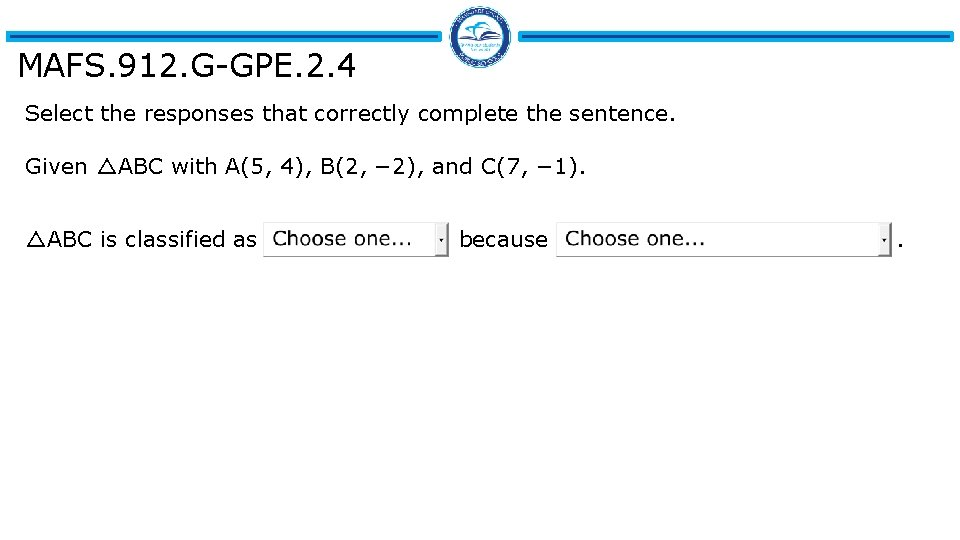MAFS. 912. G-GPE. 2. 4 Select the responses that correctly complete the sentence. Given △ABC with A(5, 4), B(2, − 2), and C(7, − 1). △ABC is classified as because .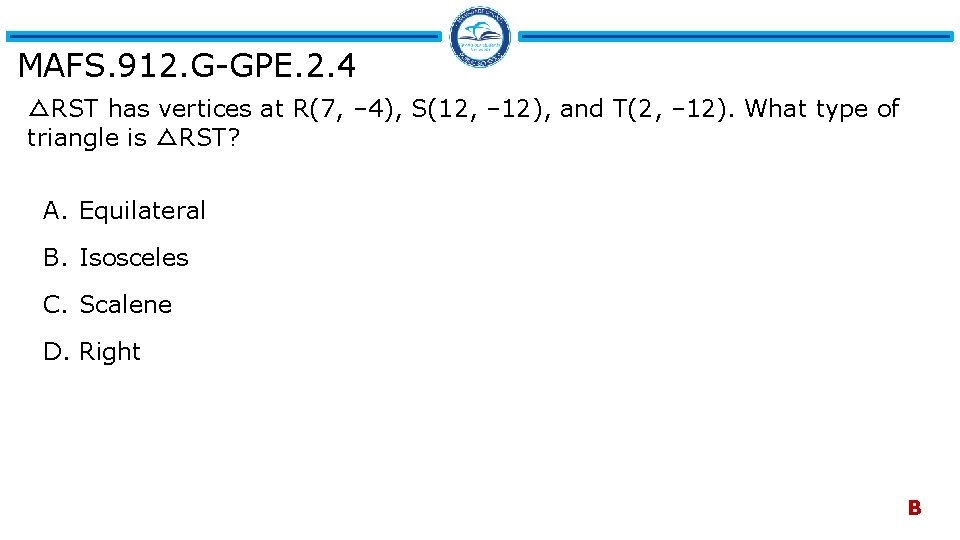MAFS. 912. G-GPE. 2. 4 △RST has vertices at R(7, – 4), S(12, – 12), and T(2, – 12). What type of triangle is △RST? A. Equilateral B. Isosceles C. Scalene D. Right B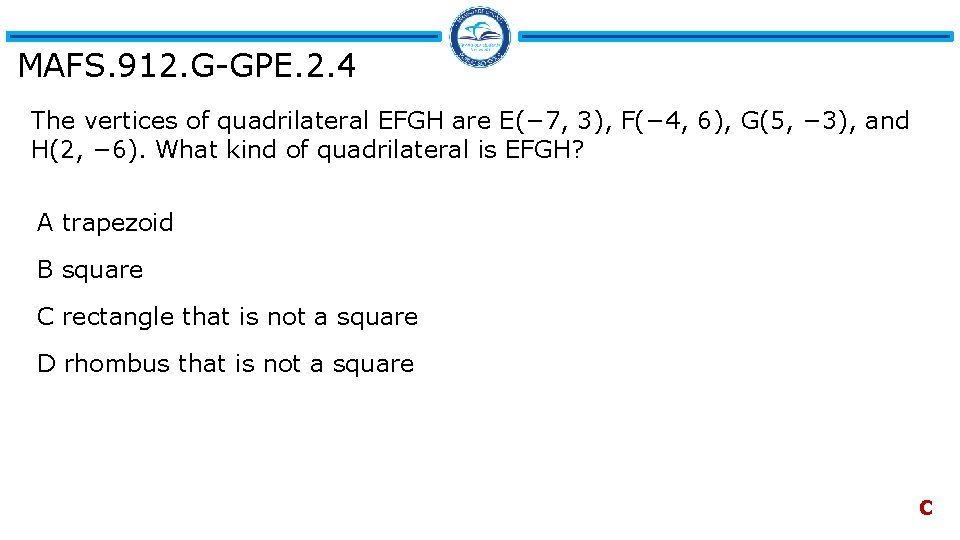MAFS. 912. G-GPE. 2. 4 The vertices of quadrilateral EFGH are E(− 7, 3), F(− 4, 6), G(5, − 3), and H(2, − 6). What kind of quadrilateral is EFGH? A trapezoid B square C rectangle that is not a square D rhombus that is not a square C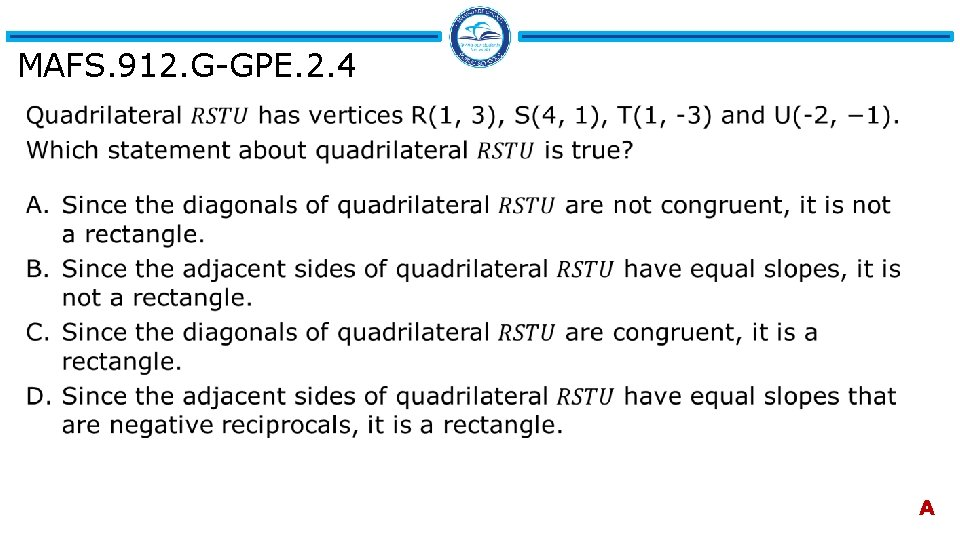MAFS. 912. G-GPE. 2. 4 AMAFS. 912. G-GPE. 2. 5 B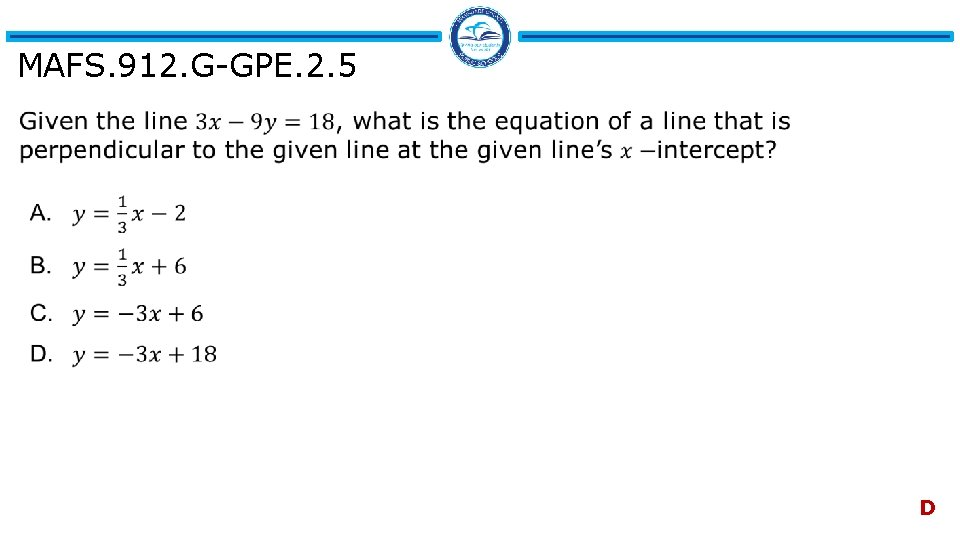MAFS. 912. G-GPE. 2. 5 D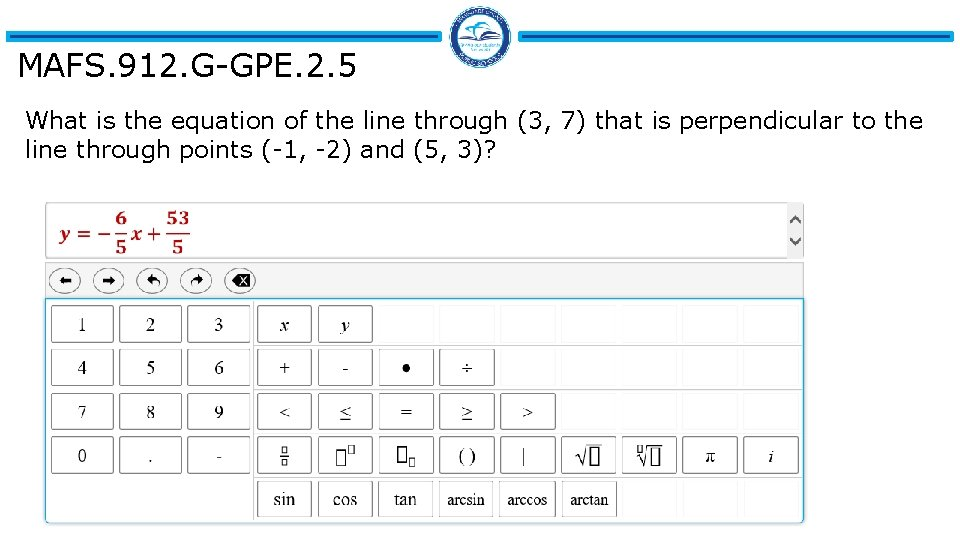MAFS. 912. G-GPE. 2. 5 What is the equation of the line through (3, 7) that is perpendicular to the line through points (-1, -2) and (5, 3)?MAFS. 912. G-GPE. 2. 5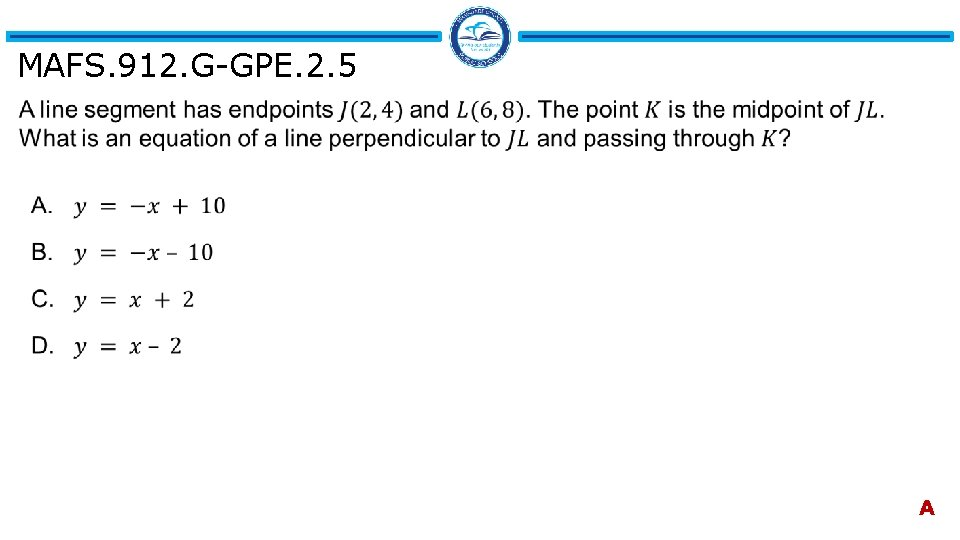MAFS. 912. G-GPE. 2. 5 A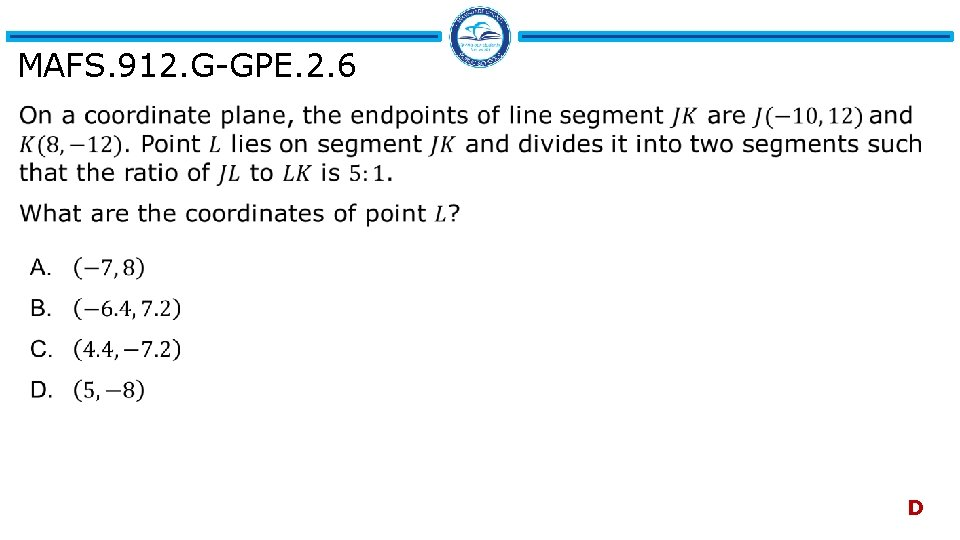MAFS. 912. G-GPE. 2. 6 DMAFS. 912. G-GPE. 2. 6 Enter your answer in the boxes. 6 0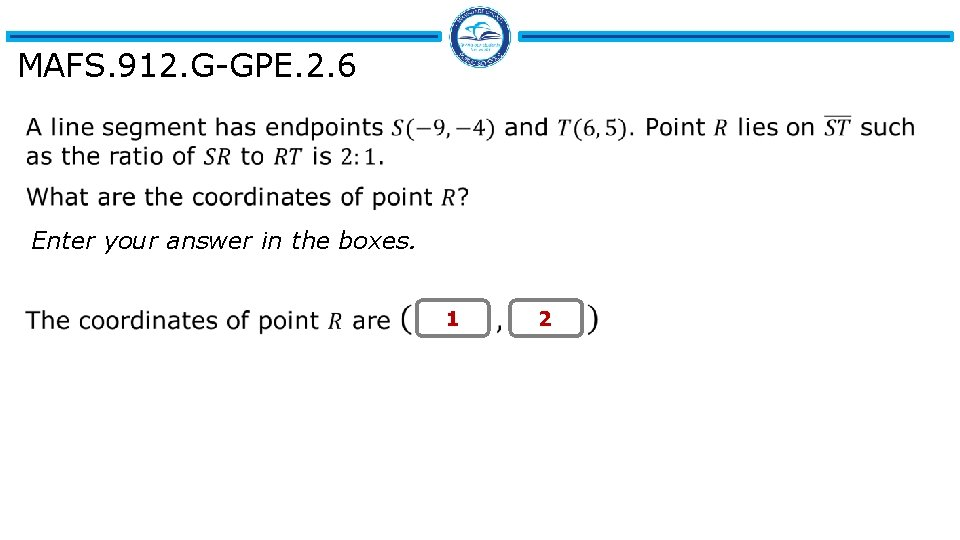MAFS. 912. G-GPE. 2. 6 Enter your answer in the boxes. 1 2MAFS. 912. G-GPE. 2. 6 3 3MAFS. 912. G-GPE. 2. 6 B, GMAFS. 912. G-GPE. 2. 7 A. 10 B. 20 C. 25 D. 50 C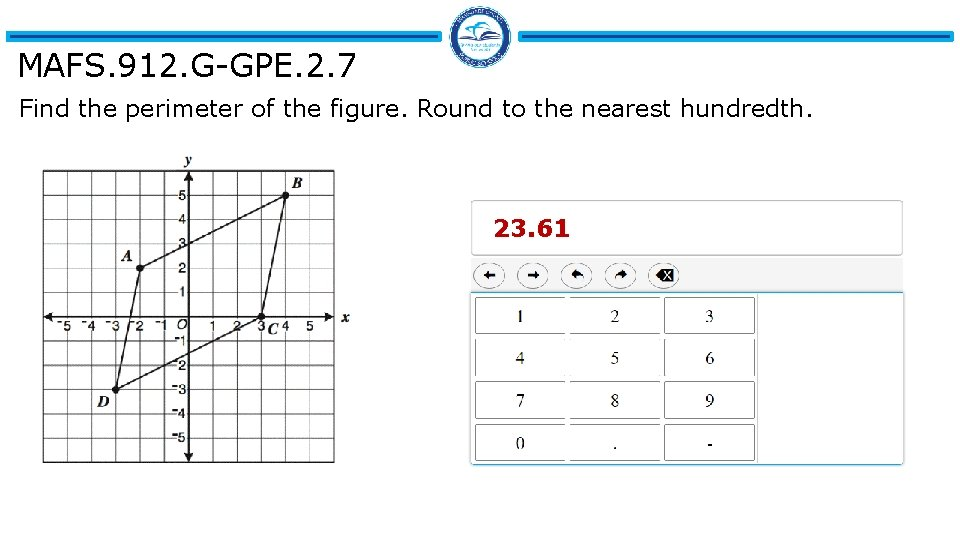MAFS. 912. G-GPE. 2. 7 Find the perimeter of the figure. Round to the nearest hundredth. 23. 61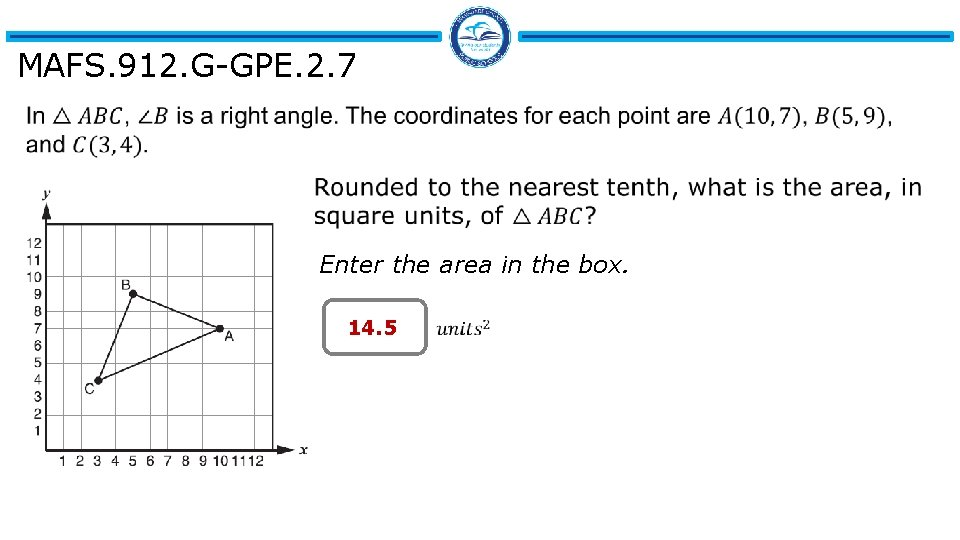MAFS. 912. G-GPE. 2. 7 Enter the area in the box. 14. 5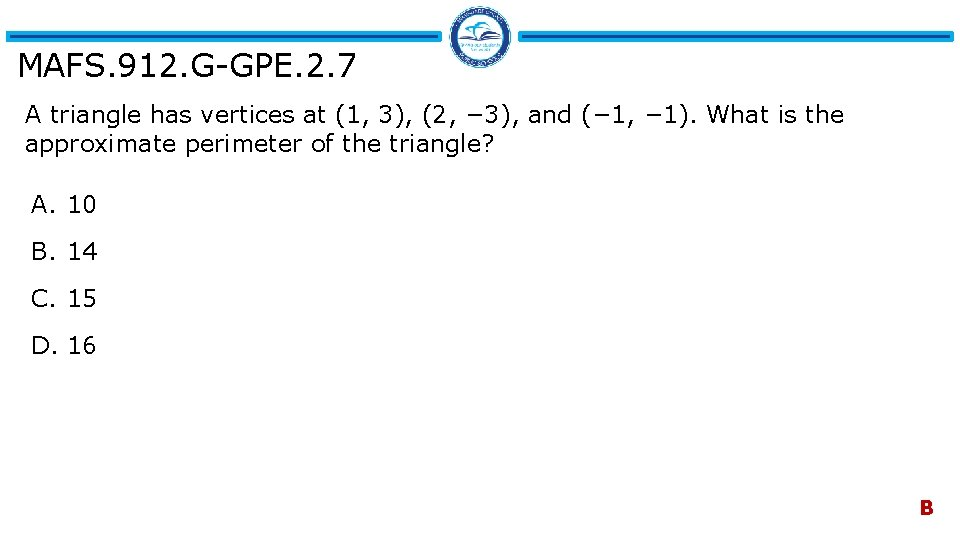MAFS. 912. G-GPE. 2. 7 A triangle has vertices at (1, 3), (2, − 3), and (− 1, − 1). What is the approximate perimeter of the triangle? A. 10 B. 14 C. 15 D. 16 BMAFS. 912. G-GPE. 2. 7 Given the polygon with vertices P(5, -1), Q(-1, -4), R(-3, 1), and S(0, 3). Find the area and the perimeter of PQRS. Round your answer to the nearest tenth. Enter your answers in the boxes. Area: Perimeter: 29 22. 1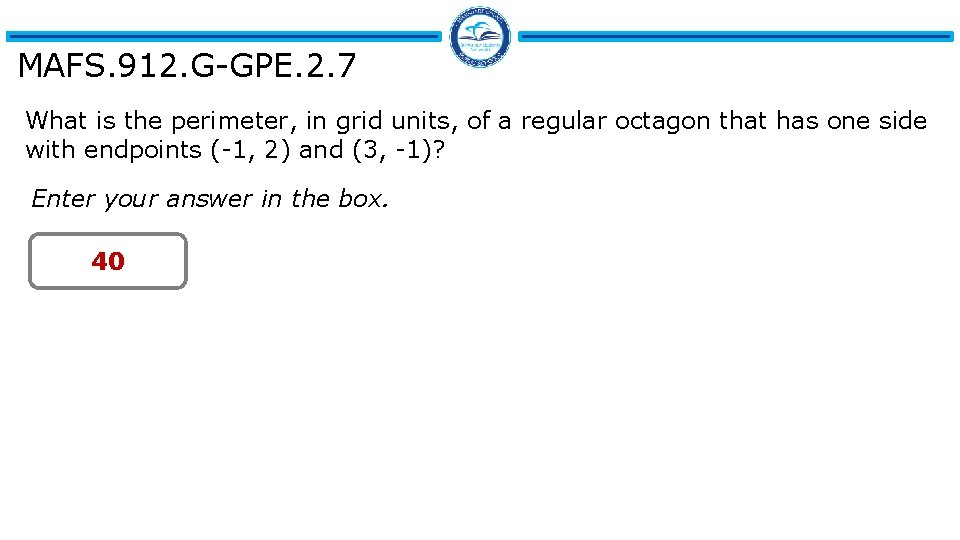MAFS. 912. G-GPE. 2. 7 What is the perimeter, in grid units, of a regular octagon that has one side with endpoints (-1, 2) and (3, -1)? Enter your answer in the box. 40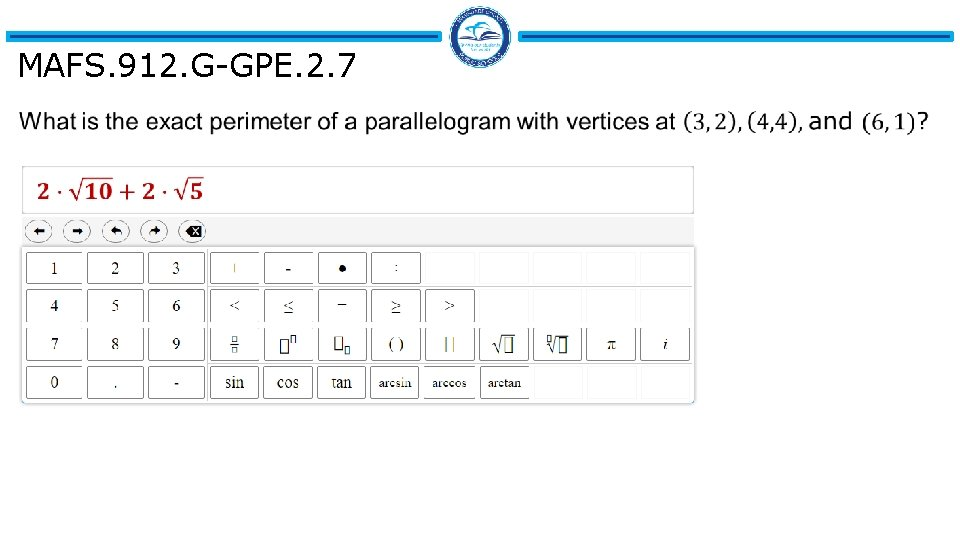MAFS. 912. G-GPE. 2. 7